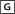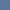# Non-Photorealistic Computer Graphics Library

[ home · search · about · links · contact · rss ] [ submit bibtex ] [ BookCite · NPR Books ]

User:

Pass:

 1963 1967 1969 1976 1977 1978 1979 1982 1983 1984 1985 1986 1987 1988 1990 1991 1992 1993 1994 1995 1996 1997 1998 1999 2000 2001 2002 2003 2004 2005 2006 2007 2008 2009 2010 2011 Authors References
Found 1 item(s) authored by "C. M. Gold".Automated contour mapping using triangular element data structures and an interpolant over each irregular triangular domain

Author(s): C. M. Gold, T.D. Charters, J. Ramsden.
Proceedings: ACM SIGGRAPH Computer Graphics, Vol. 11, No. 2, pp. 170--175, 1977.
[BibTeX] [DOI]Abstract:
Surface display techniques, including contour mapping, usually use an interpolant when data values are on a rectangular grid; but when values are irregularly located some form of assumed or estimated distance-weighting function is often used to estimate the "influence" of data points at other locations. If moving average (or some other) techniques are then applied, slopes may be zero at data points; extrema may fall at data points exclusively; or surfaces may not pass through data points. An alternative method is proposed whereby a map area is automatically divided into suitable triangular domains with a data point at each vertex. Surface estimation and contour plotting are then performed independently for each triangle using a measured or best-fit plane associated with each data point. The approach requires the utilization of three non-original aspects: 1) the derivation of a local homogeneous "area" coordinate system for any arbitrary triangle; 2) the construction of a data-structure linking each triangular domain with its three neighbouring triangles and three associated data points, and 3) the use of a "conforming" triangular finite-element interpolating function. Use of the first two of these concepts permits the economic generation and optimization of a triangular mesh from a set of data points. Optimization criteria used to define the "best" triangular partition are described in some detail, along with computer timing for this step. Use of the first and third concepts permits the interpolation of a smooth surface over the whole map area even though each triangular element is estimated and plotted independently. The requirements for a suitable interpolating function are discussed, and an interpolant is suggested that preserves elevation and slope at each data point as well as elevation and slope continuity between domains. Extrema need not be located at data points but are constrained by their associated planes. Contour line segments are produced by division of each triangle into N2 sub-triangles, elevation estimation at each resulting node, and linear interpolation and plotting withing each subtriangle. Resolution is then a function of N and local data point density. Extension of the method to higher dimensions is briefly discussed.

Visitors: 147444